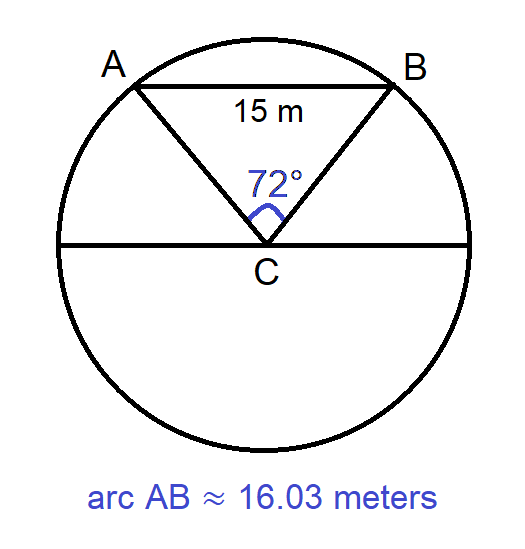# Result of the Day 2 [Problem A] Challenge of my July 2021 Math mini-contest for D.Buzz

in STEMGeeks4 months ago

## Day 2 Problem A

A curved rooftop can be represented as an arc comprising 20% of the circumference of a circle, whose endpoints are 15 meters apart. How long is the roof?

The problem has originally been posted at https://stemgeeks.net/@savvyplayer/julymathday2a.

# 16.03 meters

## Solution

The problem is under geometry and trigonometry.

• Let A and B be the endpoints of the arc, where Chord AB measures 15 meters.
• Since the arc occupies 20% of the circumference of the circle, the arc occupies 360° times 20% = 72°, so the angle of the sector inscribing the arc is also 72°.
• Let C be the center of the circle. Connecting C to A and B to form the radii of the circle, we now have an isosceles triangle ACB. The two equal angles of the triangle are (180° - 72°) / 2 = 54°.

We now have an isosceles triangle with three given angles and one given side. We can use the Law of Sines to solve for the sides of the isosceles triangle which represent the radii of the circle.

Law of Sines

## c / sin (C) = a / sin (A) = b / sin (B)

Inserting the value we have into the Law of Sines formula, we have the following partial solution:

• a / sin (54°) = 15 / sin (72°)
• a ≈ 15 * 0.8090 / 0.9511
• a ≈ 12.7598

The leg of the isosceles triangle, which is also the radius of the circle, is approximately 12.7598 meters.

Finally, to get the length of the arc which represents the length of the curved roof, we just need to get the circumference of the circle, then multiply it by 20%. The circumference of the circle is approximately 80.1719 meters, which when multiplied by 20% gives 16.0343 meters.## Winner: none

1 HIVE has been transferred to the prize pool, which will be awarded to the participant with the highest number of correctly-answered problems after all the challenge problems in this Math mini-contest have been concluded.

## Special winner: @lolxsbudoy

I have awarded 1 HIVE to @lolxsbudoy for being our special participant who answered the problem correctly. I would like to optionally invite him to show his solution for the problem.

Mentions: @jfang003, @holovision, @eturnerx (@eturnerx-dbuzz), @ahmadmanga (@ahmadmangazap), @appukuttan66, @paultactico2, @dkmathstats, and @minus-pi
Special mentions: @dbuzz, @chrisrice, @jancharlest, and @mehmetfix

Posted with STEMGeeks

Sort:

Yeyyy, thanks savvy... I got the same answer with the same solution.

4 months ago (edited)

How same our solution is might determine how much we think alike.

Wow, that's cool!

The other participants in my previous Math mini-contests used to provide their solutions too. The solutions shown are usually short but clear.

!PIZZA !LUV